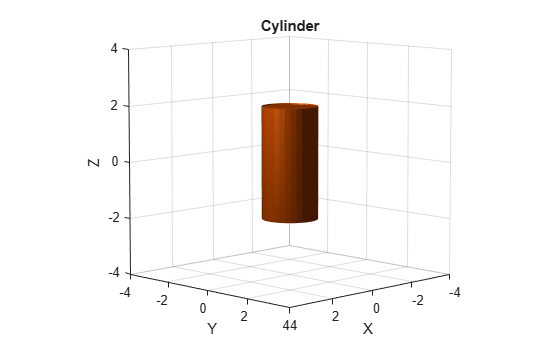# collisionCylinder

Create collision cylinder geometry

Since R2019b

## Description

Use `collisionCylinder` to create a cylinder collision geometry centered at the origin.

## Creation

### Syntax

``CYL = collisionCylinder(Radius,Length)``
``CYL = collisionCylinder(___,Pose=pose)``

### Description

example

````CYL = collisionCylinder(Radius,Length)` creates a cylinder collision geometry with a specified Radius and Length. The cylinder is axis-aligned with its own body-fixed frame. The side of the cylinder lies along the z-axis. The origin of the body-fixed frame is at the center of the cylinder.```
````CYL = collisionCylinder(___,Pose=pose)` sets the `Pose` property of the cylinder to `pose`, relative to the world frame.```

## Properties

expand all

Radius of cylinder, specified as a positive scalar. Units are in meters.

Data Types: `double`

Length of cylinder, specified as a positive scalar. Units are in meters.

Data Types: `double`

Pose of the collision geometry relative to the world frame, specified as a 4-by-4 homogeneous matrix or an `se3` object. You can change the pose after you create the collision geometry.

Note

Note that when the pose is specified as an `se3` object, the `Pose` property stores the pose as a numeric 4-by-4 matrix.

Data Types: `single` | `double`

## Object Functions

 `show` Show collision geometry `convertToCollisionMesh` Convert collision primitive geometry into collision mesh geometry `fitCollisionCapsule` Fit collision capsule around collision geometry

## Examples

collapse all

Create a cylinder collision geometry centered at the origin. The cylinder is 4 meters long with a radius of 1 meter.

```rad = 1; len = 4; cyl = collisionCylinder(rad,len)```
```cyl = collisionCylinder with properties: Radius: 1 Length: 4 Pose: [4x4 double] ```

Visualize the cylinder.

```show(cyl) title("Cylinder")```Create a homogeneous transformation that corresponds to a clockwise rotation of $\pi /4$ radians about the y-axis. Set the cylinder pose to the new matrix. Show the cylinder.

```ang = pi/4; mat = axang2tform([0 1 0 ang]); cyl.Pose = mat; show(cyl)```## Version History

Introduced in R2019b

expand all